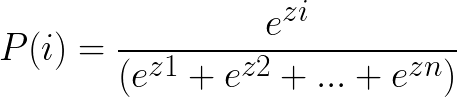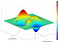# Must Know Deep Learning Concepts for Beginners — Part 1

## What is Deep Learning?

Deep Learning relates to the field of ML-based algorithms, focusing on how neurons in the brain work and applying a similar analogy to help machines learn by the use of Artificial Neural Networks.

Neural Network is a concept at the heart of Deep Learning, which can be thought of as similar to a function in a programming language. The simplest unit of Neural Network, a Perceptron, takes in inputs (like parameters of a function), runs through the process (functional steps), and finally provides a response (the output of the function).

## What do Neural Networks do?

Neural Networks generate solutions that can classify data based on the factors that affect the classification. Also, perceptrons are just an encoding of our solutions in a graphical format — depicted similar to the neurons in the brain.

# Concepts —

## 1. Classification using Linear Boundaries —

Given various data points for a linear classification 2D model, we can use a straight line to classify the data into 2 classes. This will give us a binary output i.e. is the point on the positive side of the line or the negative side.

Therefore, the equation of the boundary line / straight line will be given as —

w1 x1 + w2 x2 + b = 0 which can be represented in the vector form as
Wx + b = 0. Here, ‘W’ is the weights vector (w1, w2) for ‘x’ which is the input vector (x1, x2). The weights represent the importance of the input variable when the computation occurs inside the perceptron.

Finally, the output is ‘y’ which is the prediction w.r.t. the value of Wx + b. This tells us that if the output is 1, it’s on the positive side of the line and on the negative side if it’s 0. Thus, the prediction can be represented as —
y = { 1 if Wx + b ≥ 0
0 if Wx + b < 0 }

Now, if we have more than 2 dimensions and our perceptron is a bit more complex with n-dimensions, the boundary equation will be similar as above except our vectors will have n-values instead of 2. Although the prediction remains the same — because we’re still rooting for if the value satisfies or not. The activation function (which gives us the prediction) we’ve been using so far is the Step Function — since it outputs either 0 or 1.

In real life, we don’t build these perceptrons ourself. Instead, we provide the results, and they build themselves. To do this, we plot all the points and a model line. So, any misclassified point would want the line to move towards it, thus decreasing the error, and eventually go over to the other side so that the point is correctly classified.

To automate the process, we find new coefficients (weights) w.r.t. the learning rate and the equation of a model line and iterate over till most of the points are correctly classified.

## 2. Classification in a Non-Linear Region —

For non-linear regions, we need to generalize the Perceptron Algorithm in a way that it can be used even for curves. This is where the Error function comes in — telling us how far we are from the solution. And if we constantly take steps to decrease the error, we’ll eventually solve our problem.

Error functions should be differentiable and continuous so that even small variations can be detected. Also, continuous functions are better than discrete ones when it comes to optimizing. Therefore, we need to make predictions continuous too.

So far for the prediction, we’ve been using the Step Function. But when we move from discrete to continuous we change our Activation Function from Step function to Sigmoid Function.

The Sigmoid function instead of giving us binary output (classified or not) like the Step function, provides us with the probability of how correctly something is classified.

## 3. Softmax Function —The probability for the ith class given linear scores for n-elements.

For multi-class classification, we cannot use the Sigmoid Function because it just gives us a probability of correct classification. So for more than 2 classes, we use the Softmax Function. Given linear function scores — z1, z2, … , zn. We calculate the probability of our data being in a specific class. We turn the scores into probabilities using the Softmax Function. The probabilities of all the classes should add to 1.

## 4. One-Hot Encoding —

So far we’ve seen that all our algorithms accept numeric data. But we don’t always have numerical data. Therefore, to counter this we will come up with one variable for each class. For eg., while differentiating between 3 fruits — apple, orange, and banana, we will have one variable for each of the class. Since each of them has their corresponding column, for apple the apple variable will be 1 while the rest will be 0. This ensures that there are no unnecessary dependencies.

## 5. Maximum Likelihood —

We can use probability to make our models better. Thus, given already present models, the best out of the lot will be the one that could account for a better prediction.

Maximum Likelihood gives existing labels higher priority based on the prediction of the probability of the events that were processed. Now, we need to maximize the probability of occurrence of correct events i.e. minimizing the error.

## 6. Cross Entropy —

The definition of entropy refers to the degree of disorder or randomness and this is similar to the cross-entropy concept in DL. After calculating the probabilities of various classes, we need to calculate the overall probability of the model. Since multiplication of large dataset of probabilities ranging between 0 and 1 will give a very tiny result, we use logarithm(ln) to convert multiplication to addition.

ln(ab) = ln(a) + ln(b)

Thus, cross-entropy is defined as the sum of the negative of the logarithm of the probability. So, a low cross-entropy will mean a good model whereas bad model will have a higher cross-entropy. Therefore, we will see that correctly classified points will have smaller values and misclassified ones will have higher values. The negative of the logarithm can be thought of as errors at each point. So, correctly classified points will have smaller errors and vice versa.

Summarizing, cross-entropy tell us that if we have a few events and their probabilities, how likely will those events occur based on probability. If an event is likely to happen — it’ll have a low cross-entropy and vice versa.

## 7. Logistic Regression —

The Logistic Regression technique can be implemented as —

• Taking our data
• Picking a random model
• Calculating the error of the data using the model
• Minimizing the error, and obtaining a better model
• And then repeating the steps until we reach our goal!

We can say that our goal has changed from maximizing the classification probability to minimizing our error function.

To minimize the Error Function, we use a technique known as Gradient Descent. Given we have a multi-dimensional model, in this algorithm, we need to find the lowest point (error) in the graph. Therefore, the gradient descent algorithm helps us move from a higher point to a lower one (this can even be a local minimum rather than a global minimum).The Gradient Descent algorithm might get stuck in a local minimum even when a global minimum exists due to the surrounding points being at a higher level.

Written by

## MindOrks

#### Our community publishes stories worth reading on software development and design. Android | Machine Learning | #MakeEveryoneCode

Welcome to a place where words matter. On Medium, smart voices and original ideas take center stage - with no ads in sight. Watch
Follow all the topics you care about, and we’ll deliver the best stories for you to your homepage and inbox. Explore
Get unlimited access to the best stories on Medium — and support writers while you’re at it. Just \$5/month. Upgrade